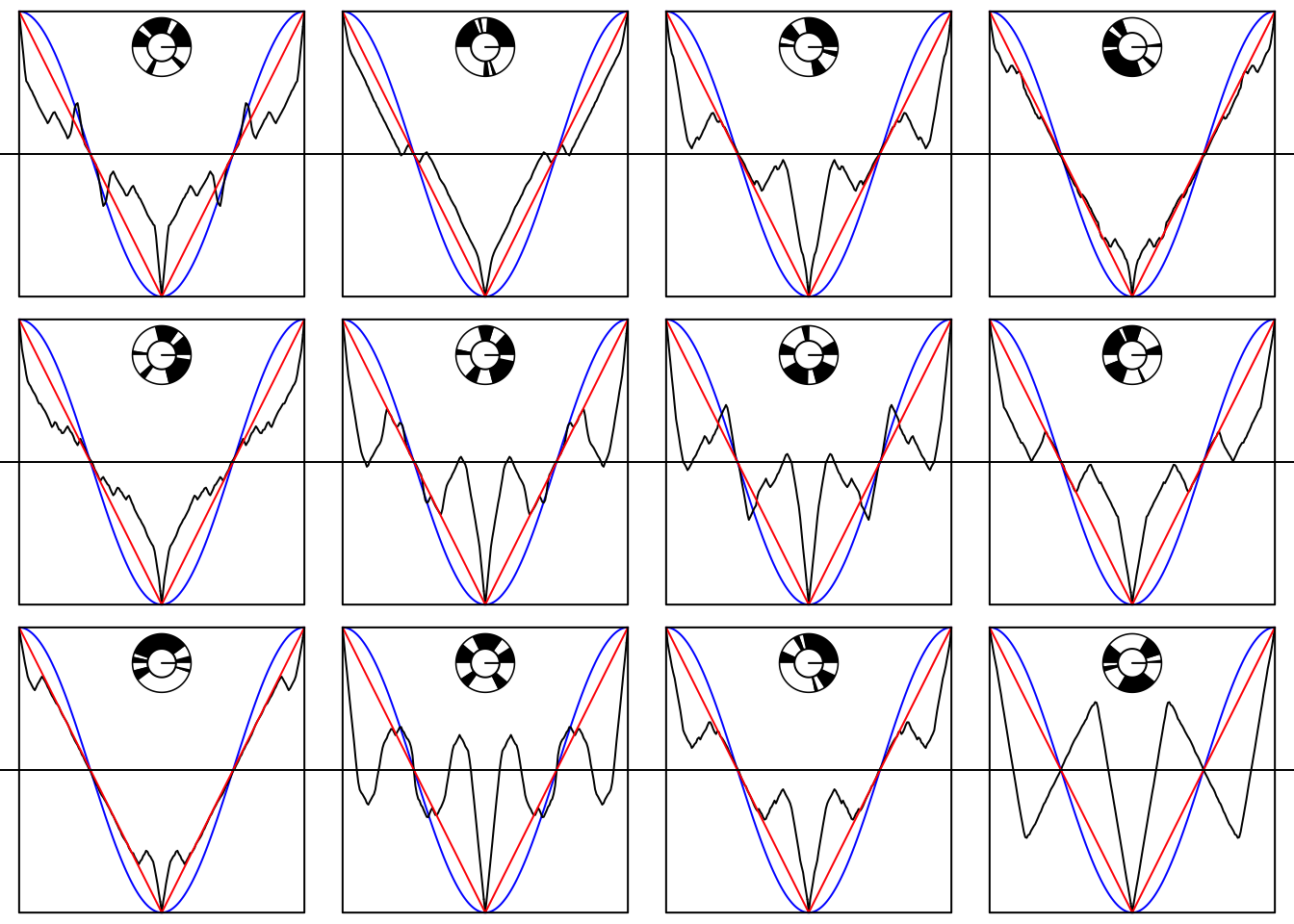# The spinning disk model of the singlet correlations
# Reference: https://arxiv.org/abs/1312.6403

# The dial at the top of each of the 12 plots is a spinning coloured disk.
# Alice and Bob each fix a point at the circumference, outside the disk.
# Think of it as their setting, or as an input.
# The settings or inputs are angles between 0 and 360 degrees.
# The disk is spun. It comes to rest.
# Alice and Bob each now have been given a "colour": "black" or "white".
# Alice converts "black" and "white" to +1, -1,
# Bob converts "black" and "white" to -1, +1;
# we call these the outputs or outcomes.
# That is called one "trial".
# Repeat many times and plot the *correlation* between the outcomes +/-1
# as a function of the difference between Alice and Bob's settings (angles).
#
# The piece-wise linear black line is what you will get
# with the coloured disk shown in the plot.
# The smooth blue line is the quantum prediction for the singlet state bla bla bla...
# and is observed in experiments in quantum optics / quantum physics.
# The red zig-zag line is what books (more precisely: conventional wisdom) will tell you
# is the best thing that can be done with a spinning disk model
# (more precisely: the best that classical physics can do).
# Conventional wisdom says that quantum correlations are stronger than classical
# because the blue negative cosine goes "outside" the red zig-zag curve.
#
# Note that the disks all have an exactly equal amount of black and white,
# and that black and white are all always exactly opposite one another.
#
# See if you can spot a couple of models exhibiting more extreme correlations,
# in some places at least, than QM does.
#
# The script runs in < 2 minutes on my laptop (MacBook Pro, 15-inch, 2018).
# You need to already have installed the Rgraphviz package
# Instructions (copy-paste two lines) are here:
# https://www.bioconductor.org/packages/release/bioc/html/Rgraphviz.html

# Normally you call it by saying: library(Rgraphviz)
suppressWarnings(suppressMessages(suppressPackageStartupMessages(library(Rgraphviz))))

oneplot <- function() {
times1 <- c(times, 1)
timesplus <- c(times1, times1 + 1, times1 + 2)
count <- function(t, d) sum(timesplus > t & timesplus <= t+d)
vecCount <- Vectorize(count)
timesplusplus <- c(0, timesplus)
numbers <- outer(data, points, vecCount)
corr <- 2*(apply(numbers%%2, 2, sum) / N) - 1
rm(numbers)
correlation <- c(corr[101:2], corr)
plot(difference / pi - 1, cos(difference), col="blue",
type="l", bty="n", ann=FALSE, xaxt="n", yaxt="n", asp = 1)
lines(difference / pi - 1, correlation, col = "black")
lines(c(-1, 0, 1), c(+1, -1, +1), col="red")
lines(c(-1, 1, 1, -1, -1),c(+1, +1, -1, -1, +1))
abline(h=0)
pieGlyph(1, 0, 0.75, labels = NA, edges = 200, radius = 0.204,
col = "black")
pieGlyph(diff(timesplusplus)[1:(2 * (nswitch + 1))] + 0.04, 0, 0.75,
labels = NA, edges = 200, radius = 0.2,
col = rep(c("black", "white"), (nswitch + 1)), border = NA)
pieGlyph(1, 0, 0.75, labels = NA, edges = 200, radius = 0.1,
col = "white")
}

points <- (0:100) / 100
difference <- pi * (c(points,  1 + points[2:101]))

N <- 10000              # 10000 is plenty; 1000 is not enough
nswitch <- 4            # Must be even!  0 is allowed, but pointless
set.seed(123456)        # Choose your own to get different pictures
# Conjecture: nswitch = 2 is pointless, too
# Empirically, 4 is best

par(mfrow = c(3, 4),oma = c(0, 0, 0, 0), mar = c(0, 0, 0, 0))    # 4 x 3 array of plots

for(i in 1:12) {
times <- sort(runif(nswitch))
data <- 2 * runif(N)
oneplot()
}